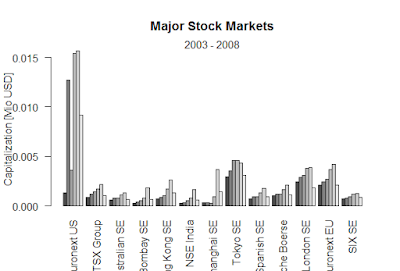Showing posts with label A model for analyzing trend such as seen in the global temperature data in is the random walk with drift model. Show all posts
Showing posts with label A model for analyzing trend such as seen in the global temperature data in is the random walk with drift model. Show all posts

## Random Walk with Drift

A model for analyzing trend such as seen in the global temperature data in  is the random walk with drift model given by xt = δ + xt−1 + wt
v = filter(w, sides=2, rep(1/3,3))
> plot.ts(v, main="moving average")
> wn = rnorm(550,0,1)
> x = filter(wn, filter=c(1,-.9), method="recursive")[-(1:50)]
> plot.ts(x, main="autoregression")
> set.seed(154)
> w = rnorm(200,0,1); x = cumsum(w)
> wd = w +.2; xd = cumsum(wd)
> plot.ts(xd, ylim=c(-5,55), main="random walk")
> lines(x); lines(.2*(1:200), lty="dashed")

### Black-Scholes formula-R

Black-Scholes formula-R > BlackScholes <- function(TypeFlag = c("c", "p"), S, X, Time, r, b, sigma) { TypeFla...